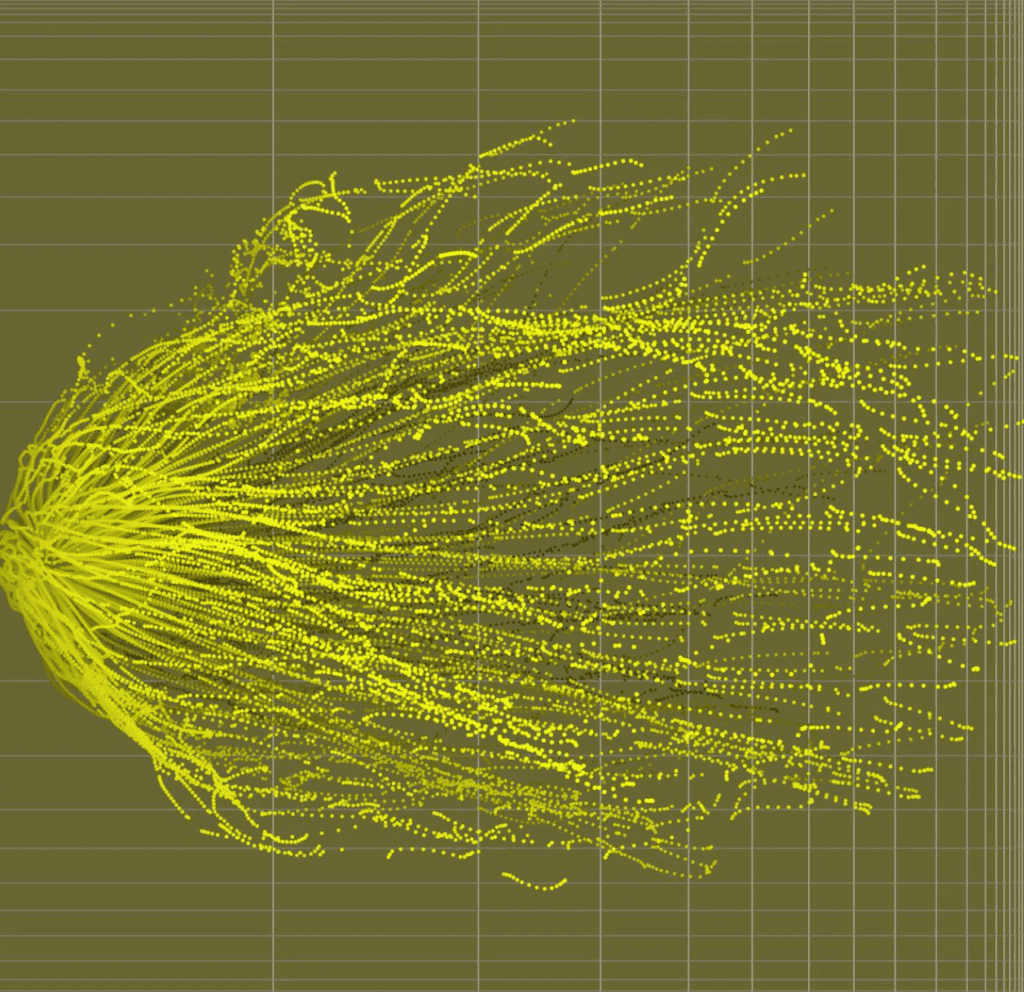MATH5835 Advanced Stochastic Processes

(1) TIME ALLOWED { 3 hours (Including time for preparing and submitting
your work)
(2) TOTAL NUMBER OF QUESTIONS { 5
(3) ANSWER ALL QUESTIONS
(4) THE QUESTIONS ARE NOT OF EQUAL VALUE
(5) TOTAL NUMBER OF MARKS { 100
(6) THIS PAPER MAY NOT BE RETAINED BY THE CANDIDATE

Problem 1.

1. [20 marks] Miss Artemis owns $K=10$ umbrellas, which are either at home or at her office in the city. She goes to work every morning and goes home every evening. If (and only if) it rains when she leaves home or work, she takes an umbrella with her (if one is available). Assume that the probability of raining when she leaves home in the morning or when she leaves work in the evening is always $p=1 / 4$, independent of previous weather conditions. Let $X_{n}$ be the number of umbrellas at home at the end of day $n$. Assume $X_{0}=K=10$.
a) [2 marks] What is the state space of the process $\left{X_{n}, n=0,1,2, \ldots\right}$.
b) [ $[5$ marks $]$ Briefly explain why $\left{X_{n}\right}$ is a Markov process, and find its transition probability matrix $P$.
c) [6 marks] Find the invariant distribution $\pi$ of $\left{X_{n}\right}$.
d) [3 marks] Is the chain reversible in equilibrium?
e) [4 marks] What is the expected number of days to wait until all the 10 umbrellas are at her home again?

Proof .

Problem 2.

$[20$ marks $]$ Let $X_{1}, X_{2}, \ldots$ be independent and let each $X_{n}$ have Poisson distribution with parameter $\lambda$.
a) $[5$ marks $]$ Show that the process
$$M_{n}=X_{1}+\cdots+X_{n}-n \lambda, \quad n \geq 1$$
is a martingale with respect to the filtration $\mathcal{F}{n}=\sigma\left(X{1}, \ldots, X_{n}\right), n=$ $1,2, \ldots$
b) $[5$ marks $]$ Let
$$\tau=\min \left{n \geq 1: X_{1}+\cdots+X_{n} \geq 2\right}$$
Show that $\tau$ is a stopping time.
c) [ $\left[\begin{array}{ll}5 \text { marks }] \text { Show }\end{array}\right.$
$$\mathbb{P}(\tau>n)=e^{-n \lambda}(1+n \lambda), \quad n=0,1, \ldots$$
d) $[5$ marks $]$ Show
$$\mathbb{E} S_{\tau}=\frac{\lambda-\lambda e^{-\lambda}+\lambda^{2} e^{-\lambda}}{\left(1-e^{-\lambda}\right)^{2}}$$
where $S_{n}=X_{1}+\cdots+X_{n}$.

\end{prob}

Proof .

Problem 3.

$[10$ marks $]$ Let $\left(M_{n}, \mathcal{F}{n}\right)$ be a martingale with $M{0}=0$ and assume that
$$\sum_{n=1}^{\infty} \mathbb{E}\left(M_{n}-M_{n-1}\right)^{2}<\infty$$
a) [5 marks] Show that there exists a random variable $M_{\infty}$ such that
$$\mathbb{P}\left(M_{n} \rightarrow M_{\infty}\right)=1, \quad \mathbb{E} M_{\infty}^{2}<\infty$$
b) [ 5 marks] Let $X_{1}, X_{2}, \ldots$ be independent, and $\mathbb{E} X_{n}^{2}<\infty$ for all $n \geq 1$. Assume also that
$$\sum_{n=1}^{\infty} \mathbb{E}\left|X_{n}\right|<\infty, \quad \sum_{n=1}^{\infty} \mathbb{E} X_{n}^{2}<\infty$$
Show that the series
$$\sum_{n=1}^{\infty} X_{n}$$
converges with probability one.

\end{prob}

Proof .

Problem 4.

$[20$ marks $]$ Let $X$ be a random variable on a probability space $(\Omega, \mathcal{F}, \mathbb{P})$ which is Poisson distributed with mean $\lambda>0 .$ Let $\mathbb{Q}$ be the set function defined by $\mathbb{Q}(A)=\mathbb{E}\left[e^{X \log \lambda-\lambda^{2}+\lambda} ; A\right]$, for $A \in \mathcal{F}$
a) [5 marks] Recall that the moment generating function of a random variable $\xi$ is defined by $M_{\xi}(t)=\mathbb{E}\left[e^{t \xi}\right]$, for $t$ in a neighbourhood of 0 where the expectation is finite. Show that the moment generating function of $X$ is given by
$$M_{X}(t)=e^{\lambda\left(e^{t}-1\right)}, \quad t \in \mathbb{R}$$
b) [ $[5$ marks $]$ Show that the set function $\mathbb{Q}$ is a probability measure on $(\Omega, \mathcal{F}) .$
c) [5 marks] Find the distribution of $X$ under the probability measure $\mathbb{Q}$.
d) [ $[5$ marks $]$ Find a probability measure $\mathbb{M}$ under which the distribution of $X$ is Poisson with mean 1 .

Proof . 用定义即可

Problem 5.

$[10$ marks $]$ Let $B_{t}, t \geq 0$ be a standard Brownian motion, $N_{t}, t \geq 0$ be a Poisson process with rate $\lambda>0$. Assume $\left{B_{t} ; t \geq 0\right}$ and $\left{N_{t} ; t \geq 0\right}$ are independent. Define $X_{t}=\sigma B_{t}+N_{t}, t \geq 0$, where $\sigma \in \mathbb{R}$.
a) $[5$ marks $]$ Show $M_{t}=\exp \left{\theta X_{t}-\frac{1}{2} \theta^{2} \sigma^{2} t+\lambda\left(1-e^{\theta}\right) t\right}, t \geq 0$ is a
martingale with respect to the filtration $\mathcal{F}{t}=\sigma\left{B{s}, N_{s} ; s \leq t\right}, t \geq 0$
b) [ $[5$ marks $]$ Solve the stochastic differential equation
$$\mathrm{d} r(t)={\theta(t)-\alpha(t) r(t)} \mathrm{d} t+\sigma(t) \mathrm{d} B(t)$$
with initial condition $r(0)=r_{0}$. [Hint: Try to find a deterministic function $A(t)$ such that $\mathrm{d}{A(t) r(t)}$ is free of $r(t) .]$

Proof .

## Stochastic Analysis Module description

Syllabus

Normal random variables and Gaussian processes; martingales; Brownian motion, stochastic integral, rules for stochastic calculus (Ito, martingale representation, Levy characterisation);applications: stochastic differential equations; martingale representation and Girsanov’s change of measure.

Prerequisites

7CCMFM01, real analysis, basic probability theory.

## Stochastic Analysis Assessment details

Assessment

2 hr written examination, class test, or alternative assessment

## Stochastic Analysis Educational aims & objectives

Aims

You will acquire a sophisticated understanding of several modern concepts and results in the theory of stochastic processes, including stochastic calculus and the theory of Brownian motion.

## Stochastic Analysis Teaching pattern

Four hours of lectures, 1 tutorial and 2 walk-in tutorials per week for the second half of Semester.

## Stochastic Analysis Suggested reading list

Suggested reading/resource (link to My Reading Lists)Stochastic Analysis代写认准UpriviateTA

BS equation代写

Categories: 数学代写